# Usupervised Anomaly Detection¶

Figure 5 in our post shows the DCGAN architecture. We’ll need to implement our discriminator, generator, data loading, and training code. I usually prefer to start with the the data loading piece - after all, without data we can’t do much! We’ll start by writing a simple script to pull the data from the MVTec website.

### 0. Let's Get Some Data¶

In [ ]:
!pip install jupyter
!pip install matplotlib
!pip install fastai
!pip install wget
!pip install kornia
!pip install opencv-python

In :
import sys, wget, tarfile, os
from pathlib import Path
import matplotlib.pyplot as plt
import numpy as np

In :
def simple_progress_bar(current, total, width=80):
sys.stdout.write("\r" + progress_message)
sys.stdout.flush()

def get_mvtech_dataset(data_dir, dataset_name):
data_dir.mkdir(exist_ok=True)
if not (data_dir/('%s.tar.xz'%dataset_name)).exists():
out=str(data_dir/('%s.tar.xz'%dataset_name)), bar=simple_progress_bar)
if not (data_dir/dataset_name).exists():
tar=tarfile.open(data_dir/('%s.tar.xz'%dataset_name))
tar.extractall(data_dir)
tar.close()


Now, which product class should we experiment with? For some reason, I just really enjoy the aesthetics of the hazelnut class - these images have a cool retro feel to them to me. This of course has nothing to do with how out model will perform - and please feel free to experiment with different classes!

In [ ]:
data_path=Path('data')
dset='hazelnut'
get_mvtech_dataset(data_path, dset)

Downloading: 90% [558080000 / 617098680] bytes

After our script runs, we can have a look at the structure of our data.

In [ ]:
list((data_path/dset).glob('*'))

Out[ ]:
[PosixPath('data/hazelnut/test'),
PosixPath('data/hazelnut/train'),
PosixPath('data/hazelnut/ground_truth')]

We're provided a train, test, and ground_truth (labels) folder, and within the train it looks like we're given 391 examples of non-defective hazelnuts:

In [ ]:
list((data_path/dset/'train').glob('*'))

Out[ ]:
[PosixPath('data/hazelnut/train/good')]
In [ ]:
im_paths=list((data_path/dset/'train'/'good').glob('*'))
im_paths[:5] #Look at first 5 paths

Out[ ]:
[PosixPath('data/hazelnut/train/good/177.png'),
PosixPath('data/hazelnut/train/good/136.png'),
PosixPath('data/hazelnut/train/good/079.png'),
PosixPath('data/hazelnut/train/good/234.png'),
PosixPath('data/hazelnut/train/good/357.png')]
In [ ]:
len(im_paths) #How many examples do we have?

Out[ ]:
391
In [ ]:
plt.imshow(plt.imread(str(im_paths)))
plt.title('One sexy hazelnut.');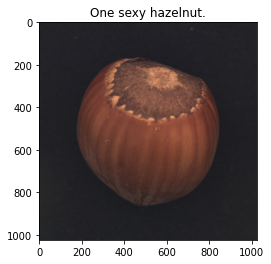Our test folder has a bit more going on, and includes examples of a 4 defect classes, and some more good examples to use in testing. We'll set aside our test set for now, and come back to it after training our GAN.

In [ ]:
list((data_path/dset/'test').glob('*'))

Out[ ]:
[PosixPath('data/hazelnut/test/print'),
PosixPath('data/hazelnut/test/crack'),
PosixPath('data/hazelnut/test/hole'),
PosixPath('data/hazelnut/test/good'),
PosixPath('data/hazelnut/test/cut')]

### 1. Data Loaders for Training¶

We’ll be using a few python libraries of note here: PyTorch, kornia, and fastai. There’s of course other fantastic tools out there like Tensorflow and Keras - PyTorch and fastai have been my go to for the last 2 years or so, especially for getting stuff up and running quickly.

In [ ]:
import kornia
from fastai.vision import *

In [ ]:
# Might need/want to supress warnings if your fastai and pytorch versions dont quite agree
import warnings
warnings.filterwarnings('ignore')


One thing I particularly like about fastai is the built in dataloader classes, called DataBunches. Let's create one for our training set. We'll create a a dataloader that will return minibatches of size 128, downsample our images to 64x64 (the resoultion used in the DCGAN paper). Our databunch will also take care of data augmentation and normalization.

In [ ]:
batch_size, im_size, channels = 64, 64, 3
data = ImageList.from_folder(data_path/dset/'train'/'good').split_none() \
.label_empty() \
.transform(tfms, size=im_size) \
.databunch(bs=batch_size) \
.normalize((0.5, 0.5))

In [ ]:
data.show_batch(figsize=(8, 8))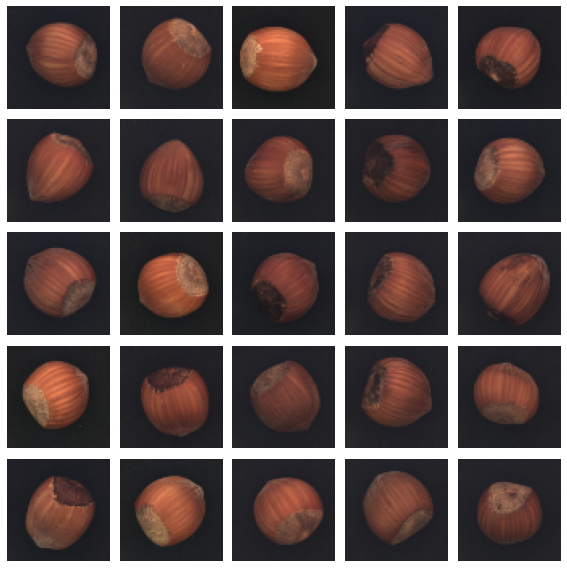Let's have a quick look at the scale of our data.

In [ ]:
x, y=data.one_batch()
plt.hist(x.numpy().ravel(),100); plt.grid(1)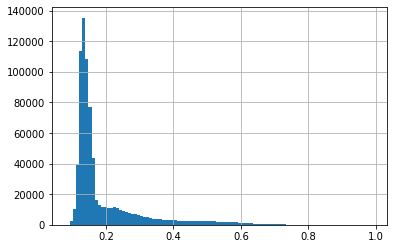### 3. Create Models in Pytorch¶

One thing I love about fastai is that it's a really light wrapper on PyTorch. There's no fastai implemenation of DCGAN, so we have to build it ourselves. Happily, we can do this in PyTorch, and still take advantage of the fastai dataloaders and training code. Before we dive in, let's figure out if we're going to be using a GPU of CPU for training. I highly recommend training this on GPU, CPU is fine for experimentation, but will take quite some time to train the full DCGAN model.

In [ ]:
import torch
import torch.nn as nn

In [ ]:
device = torch.device('cuda') if torch.cuda.is_available() else torch.device('cpu') #Do we have a GPU?
defaults.device = device
print(device)

cuda


Let's start with setting up our generator. Looking at figure X, we see that our generator is required to upsample between layers - this is generally achieved in Deep Learning models using transposed convolutional layers, also known as fractionally strided convolutional layers. There's a terrific paper on this by Vincent Dumoulin and Francesco Visin.

Note that in section 3 of the DCGAN paper, the authors call for batch normalization in the generator and discriminator, and for ReLU activations functions in every layer of the Generator, except for the output layer which uses tanh. We can make our code a bit more succint by create a conv_trans method that we can use for each layer.

In [ ]:
def conv_trans(ni, nf, ks=4, stride=2, padding=1):
return nn.Sequential(
nn.BatchNorm2d(nf),
nn.ReLU(inplace = True))


Now we can put there layers together into our generator, following the number of filters shown in figure X.

In [ ]:
G = nn.Sequential(
conv_trans(1024, 512),
conv_trans(512, 256),
conv_trans(256, 128),
nn.Tanh()).to(device)


Alright, so we have a Generator! Now, if you were paying attention in section 3.2 you may remember that the input to our generator during training is just random nosie vectors. And from these random noise vectors, our Generator is supposed to magically produce realisting looking images. Ok, so let's create a random vector:

In [ ]:
z = torch.randn(1, 100, 1, 1)


And pass it into our Generator and see what we get!

In [ ]:
fake = G(z.to(device))
plt.imshow(fake[0, 0].cpu().detach().numpy()); plt.grid(0)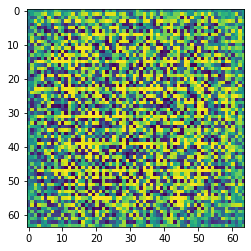Now, as you can see, our output really just looks like noise! This is of course because we haven't trained our GAN yet! When we're done this output should look like a hazelnut!

Now let's setup our discriminator. We'll use a similar patter of creating a subfunction that contains our convolution, batch normalization, and ReLU. Note here that the DCGAN authors have called for Leaky ReLU instead of ReLU.

In [ ]:
def conv(ni, nf, ks=4, stride=2, padding=1):
return nn.Sequential(
nn.BatchNorm2d(nf),
nn.LeakyReLU(0.2, inplace = True))

In [ ]:
D = nn.Sequential(
conv(channels, 128),
conv(128, 256),
conv(256, 512),
conv(512, 1024),
Flatten(),
nn.Sigmoid()).to(device)


Just as a quick sanity check, let's play with our discriminator and generator for a minute. We know that we can pass in random noise vectors into our generator and get crappy fake images out. Now, we should also be able to take these fake images and pass them into our discriminator. Let's try it!

In [ ]:
fake=G(z.to(device))
fake.shape

Out[ ]:
torch.Size([1, 3, 64, 64])
In [ ]:
D(fake)

Out[ ]:
tensor([[0.5238]], device='cuda:0', grad_fn=<SigmoidBackward>)

Just to test our thinking here - what is the meaning of this output value? Well, we create a fake image by passing in noise into our generator, and hav now passed that fake in into our discriminator, which is returning what it belives to the the probably that the image is real. Finally, just one more sanity check. As we train, we'll be passing in both real and fake data into our discriminator. We'll be getting our data from our fastai data loader:

In [ ]:
x,y=data.one_batch()

In [ ]:
x.shape

Out[ ]:
torch.Size([64, 3, 64, 64])
In [ ]:
out=D(x.to(device))

In [ ]:
plt.plot(out.detach().cpu()); plt.grid(1)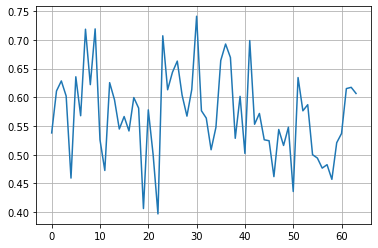So, what are we looking at here? Well, notice that the dimension of our minibatch is [128, 3, 64, 64], meaning we have 128 images we're analyzing at once in our discriminator. The outputs we've plotted are the probabilities of being real the discriminator has assigned to each image. As we can see, our results are all over the place - again, this is becuase we haven't trained anything yet. Once we're done, and effective discriminator should assign a probability close to one to each image.

### 4. Training Time¶

Alright, now that we have our data loader, discriminator, and generator set up, we can train out model! It's really helpful to have some visualization as we train, especially to see if the fake image the generator is creating look convincing. Let's start by creating a performance visualization method to show performance as we train. We'll keep track of a few key visuals while training. First, we'll choose a z_fized - 25 randomly chosen and static points in our latent space. At each visualization step, we'll pass these 25 points through our generator, and see how our fake images look. As we train, our random noise should start to be shaped into hazelnuts! Secondly, we'll plot a histogram of the pixel intensity of our fake images G(z) and compare these to our histograms of the pixel intensity values in our real images x. As we train, these distributions should look more and more similar. Finally, we'll also visualiztion our Generator and Discrimanator loss functions as we train - we should hopefully see a healthy back and forth, if either model consistenly wins, it's unlikely our fake images will look anything like real ones!

In [ ]:
from torch import optim
from tqdm import tqdm
from IPython import display
import matplotlib.gridspec as gridspec

In [ ]:
save_training_viz=True
save_dir=Path('data/exports') #Location to save training visualzations
(save_dir/'viz').mkdir(exist_ok=True, parents=True)
(save_dir/'ckpts').mkdir(exist_ok=True, parents=True)

In [ ]:
def show_progress(save=False):
'''Visualization method to see how were doing'''
plt.clf(); fig=plt.figure(0, (24, 12)); gs=gridspec.GridSpec(6, 12)
for j in range(30):
plt.imshow((kornia.tensor_to_image(fake[j])+1)/2); plt.axis('off')
ax.get_yaxis().set_ticks([]); plt.xlabel('$G(z)$', fontsize=16); plt.xlim([-1, 1])
ax.get_yaxis().set_ticks([]); plt.xlabel('$x$', fontsize=16)
plt.plot(losses, color='xkcd:goldenrod', linewidth=2); plt.plot(losses, color='xkcd:sea blue', linewidth=2);
plt.legend(['Discriminator', 'Generator'],loc=1, fontsize=16);
plt.grid(1); plt.title('Epoch = ' + str(epoch), fontsize=16); plt.ylabel('loss', fontsize=16); plt.xlabel('iteration', fontsize=16);
display.clear_output(wait=True); display.display(plt.gcf())
if save: plt.savefig(save_dir/'viz'/(str(count)+'.png'), dpi=150)


Now we'll setup our loss function and optimizers following DCGAN paper:

In [ ]:
optD = optim.Adam(D.parameters(), lr=1e-4, betas = (0.5, 0.999))
optG = optim.Adam(G.parameters(), lr=1e-4, betas = (0.5, 0.999))
criterion = nn.BCELoss()


And finally we're ready to train! On tricky thing about GANs is that no on really knows when you should stop training. One reasonable, but kinda annoying appraoch is to monitor the appearance of the generated samples while training, that's what we'll do here, while taking periodic snapshots of our weights.

In [ ]:
zero_labels = torch.zeros(batch_size).to(device)
ones_labels = torch.ones(batch_size).to(device)
losses = [[],[]]
epochs, viz_freq, save_freq, count = 10000, 100, 500, 0
z_fixed = torch.randn(batch_size, 100, 1, 1).to(device)

for epoch in range(epochs):
for i, (x,y) in enumerate(tqdm(data.train_dl)):
#Train Discriminator
requires_grad(G, False); #Speeds up training a smidge
z = torch.randn(batch_size, 100, 1, 1).to(device)
l_fake = criterion(D(G(z)).view(-1), zero_labels)
l_real = criterion(D(x).view(-1), ones_labels)
loss = l_fake + l_real
loss.backward(); losses.append(loss.item())

#Train Generator